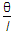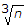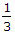# Civil Engineering - Surveying

### Exercise :: Surveying - Section 2

31.

The sum of the interior angles of a geometrical figure laid on the surface of the earth differs from that of the corresponding plane figure only to the extent of one second for every

 A. 100 sq. km of area B. 150 sq. km of area C. 200 sq. km of area D. none of these.

Explanation:

No answer description available for this question. Let us discuss.

32.

The systematic errors which persist and have regular effects in the performance of a survey operation, are due to

 A. carelessness B. faulty instrument C. inattention D. none of these.

Explanation:

No answer description available for this question. Let us discuss.

33.

It θ is the probable error of an observed bearing of a line of length l, the error over the whole length of the traverse of n lines of length l is

 A. l n B.n C. θD.θ n

Explanation:

No answer description available for this question. Let us discuss.

34.

The best method of interpolation of contours, is by

 A. estimation B. graphical means C. computation D. all of these.

Explanation:

No answer description available for this question. Let us discuss.

35.

Pick up the correct specification of Ramsden eyepiece from the following :

 A. it consists of two equal piano convex lenses B. the curved surfaces of plano-convex lenses face each other C. the two lenses are separated by a distance equal to 2/3 of the focal length of either lens. D. the distance between the diaphragm and the front lens of the eyepiece is kept equal to 1/4 th of the focal length of a lens so that rays from a point on the diaphragm enter the eye as a parallel beam E. all the above.